# Chapter 12 Probabilistic Reasoning and Bayesian Belief Networks

• Slides: 15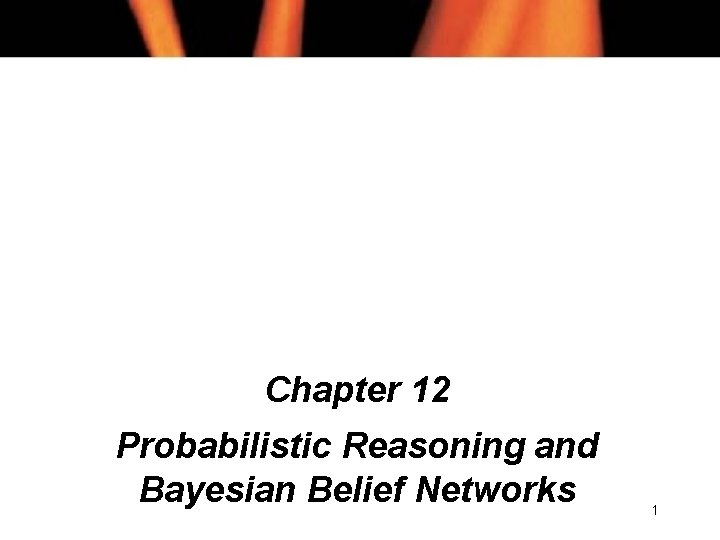Chapter 12 Probabilistic Reasoning and Bayesian Belief Networks 1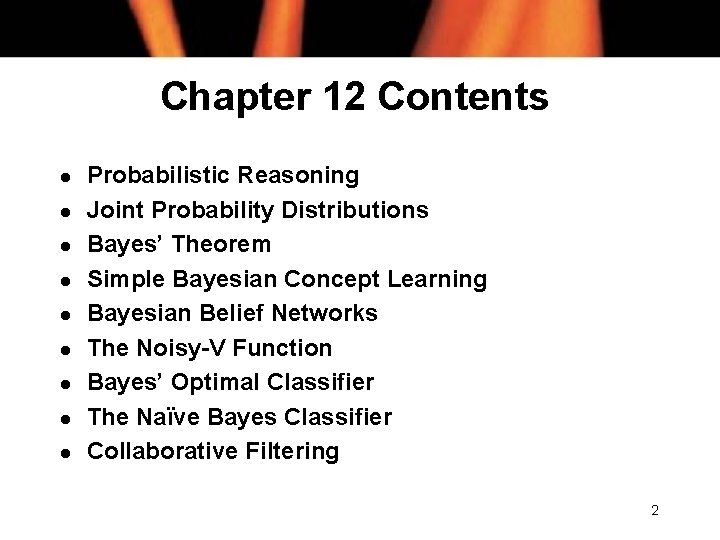Chapter 12 Contents l l l l l Probabilistic Reasoning Joint Probability Distributions Bayes’ Theorem Simple Bayesian Concept Learning Bayesian Belief Networks The Noisy-V Function Bayes’ Optimal Classifier The Naïve Bayes Classifier Collaborative Filtering 2Probabilistic Reasoning l Probabilities are expressed in a notation similar to that of predicates in FOPC: n P(S) = 0. 5 n P(T) = 1 n P(¬(A Λ B) V C) = 0. 2 l 1 = certain; 0 = certainly not 3Conditional Probability l Conditional probability refers to the probability of one thing given that we already know another to be true: l This states the probability of B, given A. 4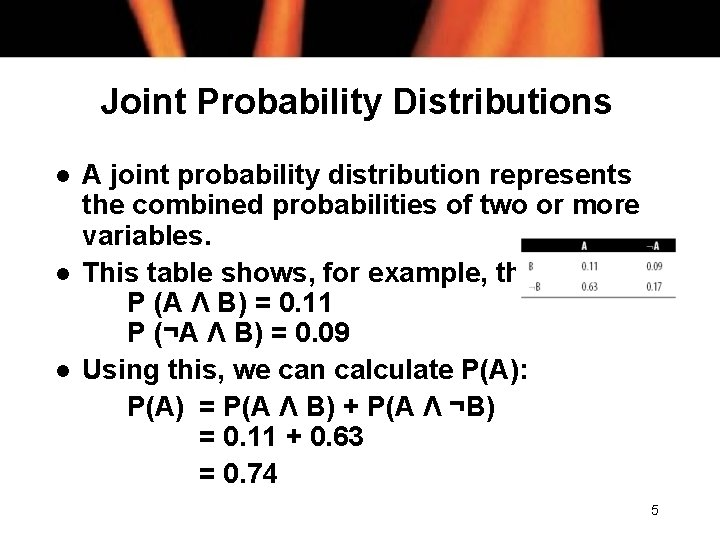Joint Probability Distributions l l l A joint probability distribution represents the combined probabilities of two or more variables. This table shows, for example, that P (A Λ B) = 0. 11 P (¬A Λ B) = 0. 09 Using this, we can calculate P(A): P(A) = P(A Λ B) + P(A Λ ¬B) = 0. 11 + 0. 63 = 0. 74 5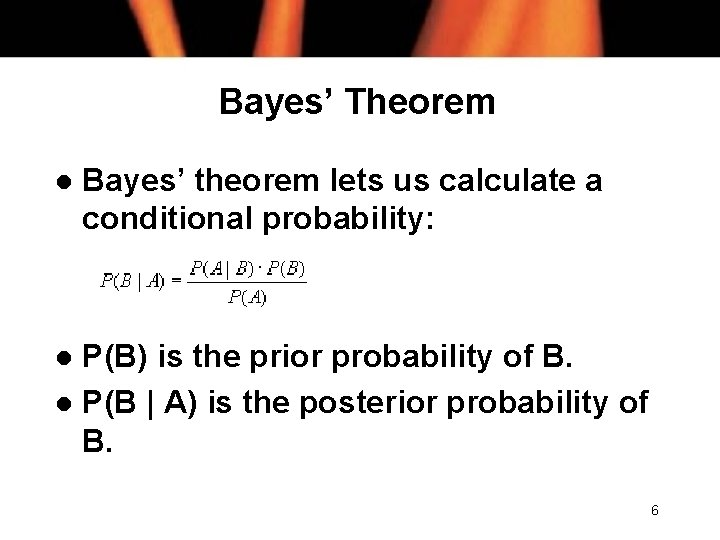Bayes’ Theorem l Bayes’ theorem lets us calculate a conditional probability: P(B) is the prior probability of B. l P(B | A) is the posterior probability of B. l 6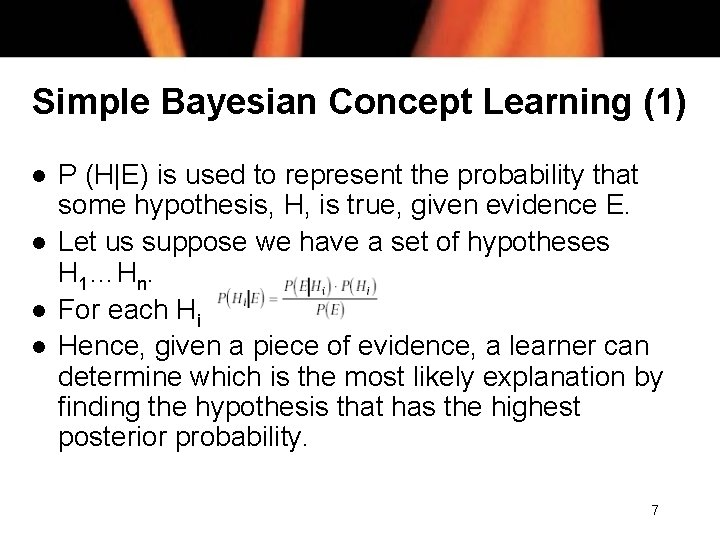Simple Bayesian Concept Learning (1) l l P (H|E) is used to represent the probability that some hypothesis, H, is true, given evidence E. Let us suppose we have a set of hypotheses H 1…Hn. For each Hi Hence, given a piece of evidence, a learner can determine which is the most likely explanation by finding the hypothesis that has the highest posterior probability. 7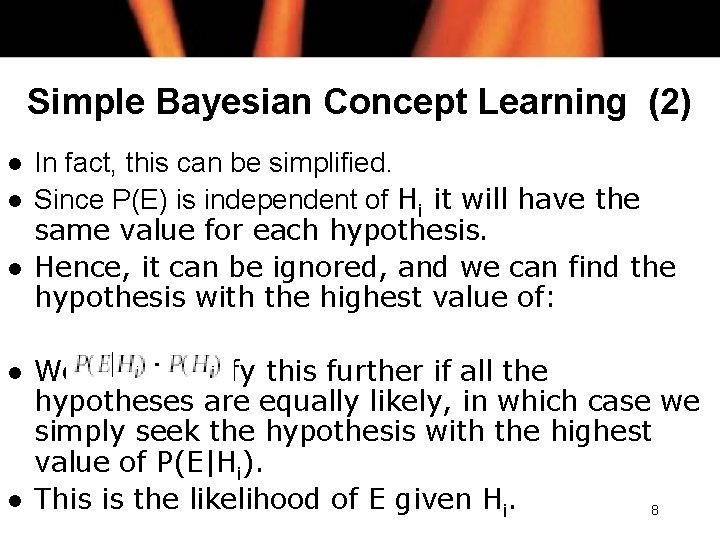Simple Bayesian Concept Learning (2) l l l In fact, this can be simplified. Since P(E) is independent of Hi it will have the same value for each hypothesis. Hence, it can be ignored, and we can find the hypothesis with the highest value of: We can simplify this further if all the hypotheses are equally likely, in which case we simply seek the hypothesis with the highest value of P(E|Hi). This is the likelihood of E given Hi. 8Bayesian Belief Networks (1) l l A belief network shows the dependencies between a group of variables. If two variables A and B are independent if the likelihood that A will occur has nothing to do with whether B occurs. C and D are dependent on A; D and E are dependent on B. The Bayesian belief network has probabilities associated with each link. E. g. , P(C|A) = 0. 2, P(C|¬A) = 0. 4 9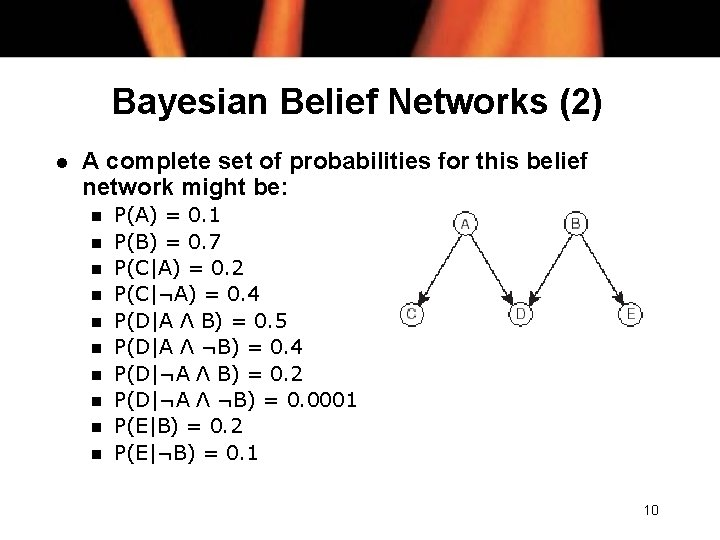Bayesian Belief Networks (2) l A complete set of probabilities for this belief network might be: n n n n n P(A) = 0. 1 P(B) = 0. 7 P(C|A) = 0. 2 P(C|¬A) = 0. 4 P(D|A Λ B) = 0. 5 P(D|A Λ ¬B) = 0. 4 P(D|¬A Λ B) = 0. 2 P(D|¬A Λ ¬B) = 0. 0001 P(E|B) = 0. 2 P(E|¬B) = 0. 1 10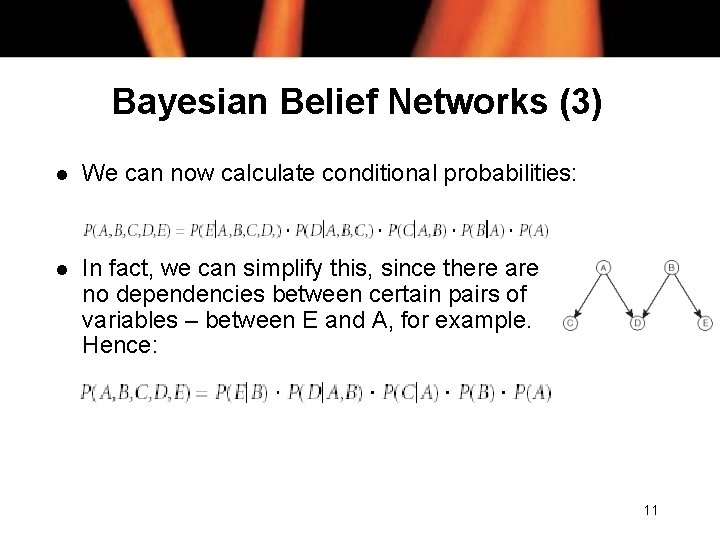Bayesian Belief Networks (3) l We can now calculate conditional probabilities: l In fact, we can simplify this, since there are no dependencies between certain pairs of variables – between E and A, for example. Hence: 11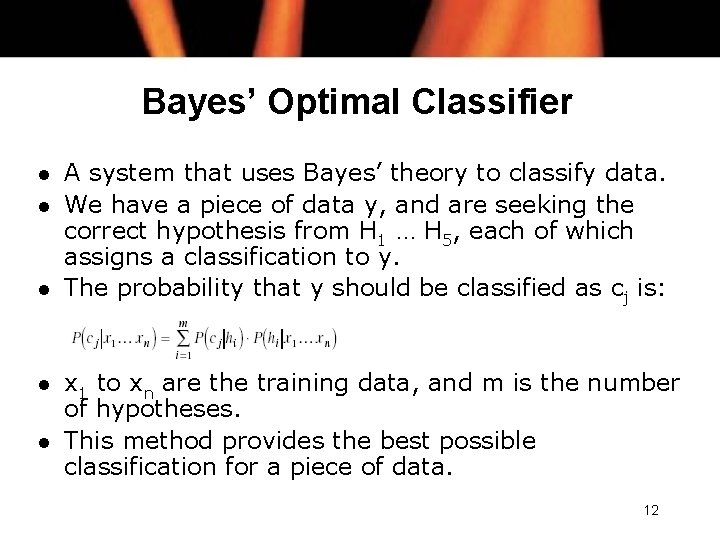Bayes’ Optimal Classifier l l l A system that uses Bayes’ theory to classify data. We have a piece of data y, and are seeking the correct hypothesis from H 1 … H 5, each of which assigns a classification to y. The probability that y should be classified as cj is: x 1 to xn are the training data, and m is the number of hypotheses. This method provides the best possible classification for a piece of data. 12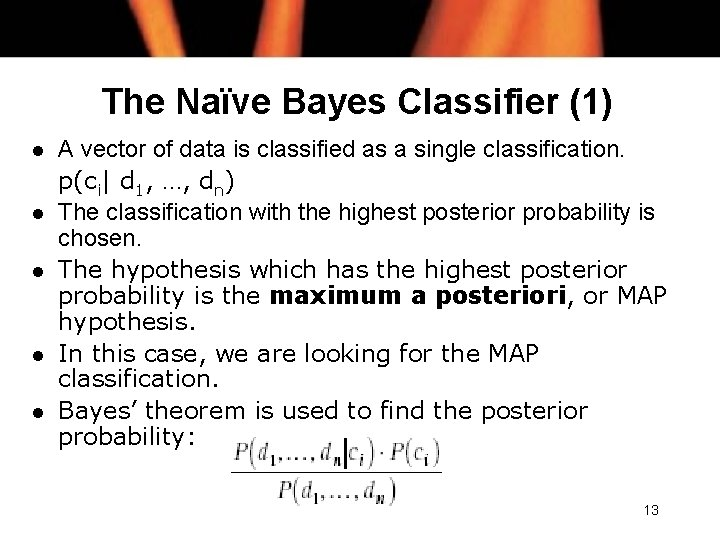The Naïve Bayes Classifier (1) l l l A vector of data is classified as a single classification. p(ci| d 1, …, dn) The classification with the highest posterior probability is chosen. The hypothesis which has the highest posterior probability is the maximum a posteriori, or MAP hypothesis. In this case, we are looking for the MAP classification. Bayes’ theorem is used to find the posterior probability: 13The Naïve Bayes Classifier (2) l since P(d 1, …, dn) is a constant, independent of ci, we can eliminate it, and simply aim to find the classification ci, for which the following is maximised: l We now assume that all the attributes d 1, …, dn are independent So P(d 1, …, dn|ci) can be rewritten as: l l The classification for which this is highest is chosen to classify the data. 14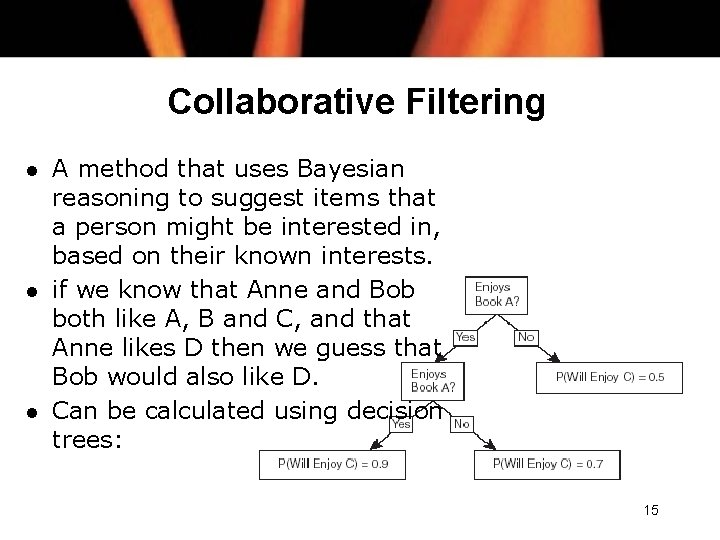Collaborative Filtering l l l A method that uses Bayesian reasoning to suggest items that a person might be interested in, based on their known interests. if we know that Anne and Bob both like A, B and C, and that Anne likes D then we guess that Bob would also like D. Can be calculated using decision trees: 15